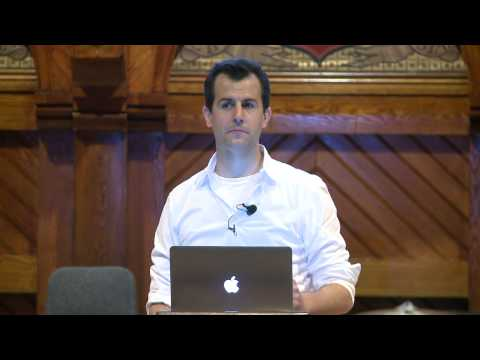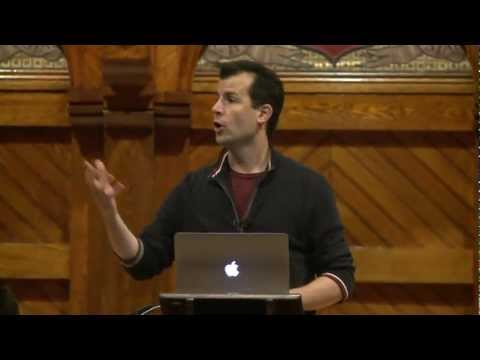# Functions

## Notes

You've probably used plenty of C functions already like printf() from the stdio library or GetInt() from CS50's library, but now we'll learn how to write our own functions and incorporate them into our code. Basically the point of functions in C is to help you to partition your code into manageable pieces.

Think of a function as a black box with a set of inputs and a single (optional) output. The rest of the program does not need to know what goes on inside the function, but the program can call the function, provide it with inputs, and use the output.

This is a bit of an oversimplification as functions are also useful for the side effects they cause. For example, you might write a function called print_instructions(), which doesn't return anything but executes a series of printf() statements.

The main reasons for using functions in programming are:

• Organization. Functions help to break up a complicated problem into more manageable subparts and help to make sure concepts flow logically into one another.

• Simplification. Smaller components are easier to design, easier to implement, and far easier to debug. Good use of functions makes code easier to read and problems easier to isolate.

• Reusability. Functions only need to be written once, and then can be used as many times as necessary, so you can avoid duplication of code.

Here's an example of the structure of a function definition. A function definition gives all the information that the compiler needs to know about a function. It describes how the function should be used, how its body should be translated into machine-readable language that the computer can execute, and what operations the code in its body will perform.

This function, cube(), takes an int as input and returns the cubed value of that int.

We'll next cover the components of a function definition in more detail.

A function definition has a header and a body.

The header always contains these parts:

• Return type. The type of value that the function will output. In our example, it is int.

• Function name. The name that is used to call this function. In our example, it is cube.

• Parameter list. The parameters are the types of arguments the function expects as input, and the names by which the function will refer to those inputs. cube() takes an int as its only argument, and this int will be referred to as input in the function's body. Note that the parameter's list can be empty, which would indicate that the function doesn't expect any arguments.

The body is the code within the curly braces that is executed when the function is called.

• It's important to remember that variables declared in the body of a function are local in scope to that function and cannot be used in other functions!

• One or more of the statements in the function's body can be return statements. A return statement ends the execution of the function and passes the return value back to the function that called it.

Let's put everything together now!

We've got our familiar main() function which calls cube() using the function's name and the argument we want to pass. Note that here the argument to cube() happens to be a variable, but more generally arguments can be constants or other expressions.

But what is that line above main() that we've highlighted in pink? This is called a function prototype. As you can see, the function prototype matches the header of the function definition except that it ends with a semicolon.

Why do we need this prototype before main()? The function prototype lets us get away with calling a function when the compiler hasn't yet seen the function's full definition (the C compiler reads from top to bottom) by providing the function’s type signature. In other words, the function prototype defines what variable types the function accepts as input and returns as output so that the compiler understands how the function should be used in main().

If you removed the prototype, you'd get a compiler error "implicit declaration of function 'cube' is invalid in C99". So you have two choices: move the definition of cube() above the definition of main() or declare a function prototype for cube() above main() as we did in this slide.

Now that you have a basic idea of how to create your own functions, let's make sure you understand how these functions are represented in memory:

At the top of the program's memory is the text segment, which contains the actual 0's and 1's that make up your program. The sections for initialized and uninitialized data are used for storing global variables if your program has any.

Most of a program's memory is reserved for the stack and heap. We won't talk about the heap today, but the stack consists of chunks of memory piled on top of each other. Just like the stack of trays in the dining hall, the stack of a program requires that the one on top be taken off before any of the others can be taken off.

Let's zoom in on the stack.

The memory at the bottom of the stack is first used for main(). Any functions that main() calls have their memory stacked on top, so we see our function cube() right above main(). As functions return, their chunks of memory are popped off the stack.

The important function implication for us is that when main() calls cube(), cube() creates copies of the variables passed to it which are stored in cube()'s stack frame. We'll see in the next example why this is such a big deal.

This program attempts to swap the values of x and y.

Let's think through the logic of that swap function:

• Take the value of a and store it in a temporary variable tmp.
• Store the value of b in a.
• Store the value of tmp in b.

Seems perfectly reasonable, right?

Why doesn't this work? Well, swap() has made copies of x and y in a different stack frame and is calling those copies a and b. Whatever operations swap() performs on a and b have no bearing on our original x and y variables in main()'s stack frame.

Discuss ways to fix this program!## Slides ( / )## Accumulating Interest

Prerequisites:

Implement a function with prototype

``double accumulate_interest(double balance, double rate);``

that takes two `double` s as input — a bank balance and an annual interest rate — and outputs an updated balance after 1 year of interest has accrued.

``````jharvard@run.cs50.net (~): ./a.out
Starting balance: 100
Annual interest rate: 0.05
Updated balance: 105.00``````

Try out some pseudocode here!
```#include <cs50.h>
#include <stdio.h>

// TODO: function prototype

int main(void)
{
printf("Starting balance: ");
double start = GetDouble();
printf("Annual interest rate: ");
double interest = GetDouble();
double updated = // TODO: call your function
printf("Updated balance: %.2f\n", updated);
}

// TODO: function definition
```

## Videos#### Monday, Week 2

An introduction to functions#### Wednesday, Week 2

Functions, continued#### Nate's Functions Short

Nate gives a general overview